# 2-sphere in Euclidean space

Arbitrary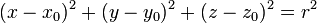$(x - x_0)^2 + (y - y_0)^2 + (z - z_0)^2 = r^2$$(x_0,y_0,z_0)$ are the coordinates of the center and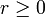$r \ge 0$ is the radius of the sphere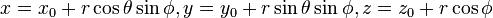$x = x_0 + r \cos \theta \sin \phi, y = y_0 + r \sin \theta \sin \phi, z = z_0 + r \cos \phi$$\theta$ and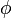$\phi$ play roles analogous to the azimuthal and polar angles. See spherical polar coordinates.
Up to translations, i.e., given any 2-sphere in Euclidean space, we can do a translation and bring it into this form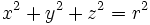$x^2 + y^2 + z^2 = r^2$$r \ge 0$ is the radius. We have used a translation to move the center of the sphere to the origin.$x = r \cos \theta \sin \phi, y = r \sin \theta \sin \phi, z = r \cos \phi$.$\theta$ and$\phi$ play roles analogous to the azimuthal and polar angles. See spherical polar coordinates.
Up to all rigid motions (translations, rotations, reflections)$x^2 + y^2 + z^2 = r^2$$r \ge 0$ is the radius. We have used a translation to move the center of the sphere to the origin. Since the sphere has rotational and reflection symmetry, allowing freedom of rotation does not result in any simplification of the equation.$x = r \cos \theta \sin \phi, y = r \sin \theta \sin \phi, z = r \cos \phi$.$\theta$ and$\phi$ play roles analogous to the azimuthal and polar angles. See spherical polar coordinates.
Up to all similarity transformations (transformations, rotations, reflections, scaling)$x^2 + y^2 + z^2 = 1$ no parameters any more. This is the unit 2-sphere centered at the origin.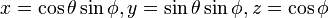$x = \cos \theta \sin \phi, y = \sin \theta \sin \phi, z = \cos \phi$.$\theta$ and$\phi$ play roles analogous to the azimuthal and polar angles. See spherical polar coordinates.